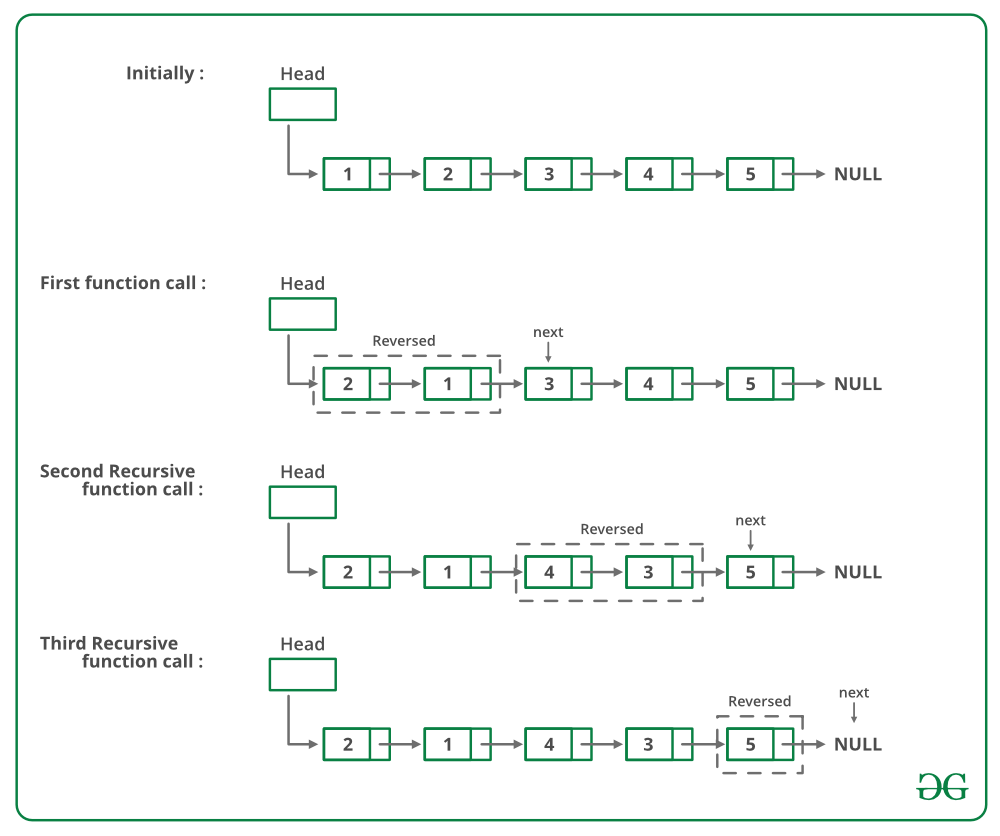# C Program For Pairwise Swapping Elements Of A Given Linked List

• Last Updated : 15 Dec, 2021

Given a singly linked list, write a function to swap elements pairwise.

```Input: 1->2->3->4->5->6->NULL Output: 2->1->4->3->6->5->NULL

Input: 1->2->3->4->5->NULL Output: 2->1->4->3->5->NULL

Input: 1->NULL Output: 1->NULL
```

For example, if the linked list is 1->2->3->4->5 then the function should change it to 2->1->4->3->5, and if the linked list is then the function should change it to.

METHOD 1 (Iterative):
Start from the head node and traverse the list. While traversing swap data of each node with its next node’s data.
Below is the implementation of the above approach:

## C

 `/* C program to pairwise swap elements  ` `   ``in a given linked list */` `#include ` `#include ` ` `  `// A linked list node  ` `struct` `Node  ` `{ ` `    ``int` `data; ` `    ``struct` `Node* next; ` `}; ` ` `  `/* Function to swap two integers  ` `   ``at addresses a and b */` `void` `swap(``int``* a, ``int``* b); ` ` `  `/* Function to pairwise swap elements  ` `   ``of a linked list */` `void` `pairWiseSwap(``struct` `Node* head) ` `{ ` `    ``struct` `Node* temp = head; ` ` `  `    ``/* Traverse further only if there  ` `       ``are at-least two nodes left */` `    ``while` `(temp != NULL &&  ` `           ``temp->next != NULL)  ` `    ``{ ` `        ``/* Swap data of node with its  ` `           ``next node's data */` `        ``swap(&temp->data,  ` `             ``&temp->next->data); ` ` `  `        ``// Move temp by 2 for the  ` `        ``// next pair  ` `        ``temp = temp->next->next; ` `    ``} ` `} ` ` `  `// UTILITY FUNCTIONS  ` `// Function to swap two integers  ` `void` `swap(``int``* a, ``int``* b) ` `{ ` `    ``int` `temp; ` `    ``temp = *a; ` `    ``*a = *b; ` `    ``*b = temp; ` `} ` ` `  `/* Function to add a node at the  ` `   ``beginning of Linked List */` `void` `push(``struct` `Node** head_ref, ``int` `new_data) ` `{ ` `    ``// Allocate node  ` `    ``struct` `Node* new_node =  ` `           ``(``struct` `Node*)``malloc``(``sizeof``(``struct` `Node)); ` ` `  `    ``// Put in the data  ` `    ``new_node->data = new_data; ` ` `  `    ``// Link the old list off the new node  ` `    ``new_node->next = (*head_ref); ` ` `  `    ``// Move the head to point to the  ` `    ``// new node  ` `    ``(*head_ref) = new_node; ` `} ` ` `  `/* Function to print nodes in a  ` `   ``given linked list */` `void` `printList(``struct` `Node* node) ` `{ ` `    ``while` `(node != NULL)  ` `    ``{ ` `        ``printf``(``"%d "``, node->data); ` `        ``node = node->next; ` `    ``} ` `} ` ` `  `// Driver code ` `int` `main() ` `{ ` `    ``struct` `Node* start = NULL; ` ` `  `    ``/* The constructed linked list is:  ` `       ``1->2->3->4->5 */` `    ``push(&start, 5); ` `    ``push(&start, 4); ` `    ``push(&start, 3); ` `    ``push(&start, 2); ` `    ``push(&start, 1); ` ` `  `    ``printf``( ` `    ``"Linked list before calling pairWiseSwap()"``); ` `    ``printList(start); ` ` `  `    ``pairWiseSwap(start); ` ` `  `    ``printf``( ` `    ``"Linked list after calling pairWiseSwap()"``); ` `    ``printList(start); ` ` `  `    ``return` `0; ` `} `

Output:

```Linked list before calling pairWiseSwap()
1 2 3 4 5
Linked list after calling pairWiseSwap()
2 1 4 3 5 ```

Time complexity: O(n)

METHOD 2 (Recursive):
If there are 2 or more than 2 nodes in Linked List then swap the first two nodes and recursively call for rest of the list.
Below image is a dry run of the above approach:Below is the implementation of the above approach:

## C

 `/* Recursive function to pairwise swap  ` `   ``elements of a linked list */` `void` `pairWiseSwap(``struct` `node* head) ` `{ ` `    ``/* There must be at-least two nodes  ` `       ``in the list */` `    ``if` `(head != NULL && head->next != NULL)  ` `    ``{ ` `        ``/* Swap the node's data with data  ` `           ``of next node */` `        ``swap(head->data, head->next->data); ` ` `  `        ``/* Call pairWiseSwap() for rest of  ` `           ``the list */` `        ``pairWiseSwap(head->next->next); ` `    ``} ` `} `

Time complexity: O(n)
The solution provided there swaps data of nodes. If data contains many fields, there will be many swap operations. See this for an implementation that changes links rather than swapping data.

Please refer complete article on Pairwise swap elements of a given linked list for more details!

My Personal Notes arrow_drop_up
Recommended Articles
Page :